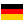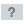# mtv.de: MTV Germany | Neue Musikvideos, alle Shows, unterhaltsame News und Popkultur

Der Ort für erstklassige Unterhaltung und für geskriptete als auch nicht-geskriptete Serien.

• 55/100

Normal result
• 6

Successful tests
• 2

Failed tests
• First response

707 ms

3.2 sec

• Page rendered

829 ms

4.7 sec

Visit mtv.de now to see the best up-to-date MTV content for Germany and also check out these interesting facts you probably never knew about mtv.de

We analyzed Mtv.de page load time and found that the first response time was 707 ms and then it took 4 sec to load all DOM resources and completely render a web page. This is a poor result, as 60% of websites can load faster.

## Page optimization

•  HTML 129.5 kB Images 2.1 MB Javascripts 596.9 kB CSS 667.9 kB

In fact, the total size of Mtv.de main page is 3.5 MB. This result falls beyond the top 1M of websites and identifies a large and not optimized web page that may take ages to load. 80% of websites need less resources to load and that’s why Accessify’s recommendations for optimization and resource minification can be helpful for this project. Images take 2.1 MB which makes up the majority of the site volume.

• Original 129.5 kB
• After minification 128.9 kB
• After compression 32.8 kB

HTML optimization

HTML content can be minified and compressed by a website’s server. The most efficient way is to compress content using GZIP which reduces data amount travelling through the network between server and browser. HTML code on this page is well minified. It is highly recommended that content of this web page should be compressed using GZIP, as it can save up to 96.7 kB or 75% of the original size.

• Original 2.1 MB
• After optimization 1.8 MB

Image optimization

Image size optimization can help to speed up a website loading time. The chart above shows the difference between the size before and after optimization. Obviously, MTV needs image optimization as it can save up to 312.8 kB or 15% of the original volume. The most popular and efficient tools for JPEG and PNG image optimization are Jpegoptim and PNG Crush.

• Original 596.9 kB
• After minification 581.5 kB
• After compression 189.9 kB

JavaScript optimization

It’s better to minify JavaScript in order to improve website performance. The diagram shows the current total size of all JavaScript files against the prospective JavaScript size after its minification and compression. It is highly recommended that all JavaScript files should be compressed and minified as it can save up to 407.0 kB or 68% of the original size.

• Original 667.9 kB
• After minification 652.6 kB
• After compression 97.2 kB

CSS optimization

CSS files minification is very important to reduce a web page rendering time. The faster CSS files can load, the earlier a page can be rendered. Mtv.de needs all CSS files to be minified and compressed as it can save up to 570.7 kB or 85% of the original size.

### Network requests diagram

• www.mtv.de
• application-mtv-d172d61ae4c7b7e7c36f4b35e005c565.css
• 960x300_
• 940x126
• 940x126
• 940x126
• iam.js
• 1895040060.js
• application_mtv-906c67ebe3c3d199d8d9fc98a5e8651b.js
• coda.html
• background-tile-a018323a1affaf142a7b8e03431e1844.gif
• mtv_logo-aa521e69c1c93fb9a1ed0e553577f866.svg
• spinner-3a2651b7e274066d61fb65b7dab2d833.gif
• OpenSans-Regular-40f3cc25f7284b35caa72e9567b8edf1.woff
• OpenSans-Bold-786732f1fdc4ca4121a8fa314663433a.woff
• viacom_icons-webfont-9e6b023baec26ab8b1d0de91b26ed322.svg
• OpenSans-ExtraBoldItalic-8b8c885e2ab5d36966dde27df7fe2063.woff
• OpenSans-Italic-8aa0a99100d020b9ecf9350b12c6b7db.woff
• Context
• ga.js
• mods.html
• beacon.js
• ;sz=6x6;tile=0;dcove=d;ord=382464537769556030
• analytics.js
• rc
• __utm.gif
• __utm.gif
• __utm.gif
• __utm.gif
• __utm.gif
• __utm.gif
• ;ord=1456747923243
• spinner-39e22b9d6c74d9c111d91856bcceab27.svg
• sessions.json
• bcn
• 960x300_
• collect
• tx.io
• ;a1=9;a10=9;a11=9;a12=9;a13=9;a14=9;a15=9;a16=9;a17=9;a18=9;a2=9;a20=9;a21=9;a22=9;a3=9;a4=9;a5=9;a6=9;a7=9;a8=9;a9=9;d1=2;d10=1;d11=1;d12=1;d2=1;d3=1;d4=3;d7=2;d8=2;d9=3;g1=1;g2=1;g3=0;g4=0;g5=0;i1=9;i10=9;i11=9;i12=9;i13=3;i14=9;i15=4;i16=9;i17=9;i18=9;i20=9;i21=4;i23=4;i26=9;i27=9;i28=3;i29=9;i3=3;i36=9;i42=3;i44=4;i46=4;i48=9;i8=9;i9=3;n1=1;n2=1;n3=0;n4=0;n5=1;n6=0;n7=0;n8=1;n9=0;f1=1;h1=0;h2=0;h3=0;h4=0;h5=0;h6=0;h7=0;h8=1;h9=0;h10=1;h11=0;h12=1;h13=1;h14=0;h15=0;h16=1;h17=1;c1=0;c2=9;sz=728x90;ord=382464537769556030
• collect
• 306x172_
• 306x172_
• 465x260_
• 306x356_
• 306x356_
• 306x172_
• 306x172_
• 465x260_
• 465x260_
• 306x356_
• 306x172_
• 306x172_
• 306x172_
• 306x172_
• ga-audiences
• s48059718492440
• 10764.js
• lidar.js
• tx.io
• sync
• all.js
• 327956-2.js
• ;ord=8018531572989539893;dc_lat=;dc_rdid=;tag_for_child_directed_treatment=
• dt
• ;ord=8018531572989539893;dc_lat=;dc_rdid=;tag_for_child_directed_treatment=
• dtc
• 15CY_AUTO_IN_MARKET_STATIC_R1_728x90.jpg
• sbhK2lTE.js
• cTrvNaRi.html
• MdyApZkAHG2-UELdOwjNjjFZXSz-CGj4o1JtDR7aGgs.js
• xd_arbiter.php
• xd_arbiter.php
• mon
• ddc.htm
• emily.html
• emily.html
• ;a1=9;a10=9;a11=9;a12=9;a13=9;a14=9;a15=9;a16=9;a17=9;a18=9;a2=9;a20=9;a21=9;a22=9;a3=9;a4=9;a5=9;a6=9;a7=9;a8=9;a9=9;d1=2;d10=1;d11=1;d12=1;d2=1;d3=1;d4=3;d7=2;d8=2;d9=3;g1=1;g2=1;g3=0;g4=0;g5=0;i1=9;i10=9;i11=9;i12=9;i13=3;i14=9;i15=4;i16=9;i17=9;i18=9;i20=9;i21=4;i23=4;i26=9;i27=9;i28=3;i29=9;i3=3;i36=9;i42=3;i44=4;i46=4;i48=9;i8=9;i9=3;n1=1;n2=1;n3=0;n4=0;n5=1;n6=0;n7=0;n8=1;n9=0;f1=1;h1=0;h2=0;h3=0;h4=0;h5=0;h6=0;h7=0;h8=1;h9=0;h10=1;h11=0;h12=1;h13=1;h14=0;h15=0;h16=1;h17=1;c1=0;c2=9;sz=300x250;ord=382464537769556030
• dt
• tap.php
• tap.php
• sync
• 327956-15.js

Our browser made a total of 86 requests to load all elements on the main page. We found that 15% of them (13 requests) were addressed to the original Mtv.de, 22% (19 requests) were made to Images.mtvnn.com and 10% (9 requests) were made to Google-analytics.com. The less responsive or slowest element that took the longest time to load (707 ms) belongs to the original domain Mtv.de.

Requests

The browser has sent 78 CSS, Javascripts, AJAX and image requests in order to completely render the main page of MTV. We recommend that multiple CSS and JavaScript files should be merged into one by each type, as it can help reduce assets requests from 27 to 1 for JavaScripts and as a result speed up the page load time.

27

Javascripts

43

Images

1

CSS

7

AJAX

78

All

Possible request optimization

1

Javascripts

27

Images

1

CSS

7

AJAX

42

Optimized

36

All

Normal result

Mtv.de uses IP address which is currently shared with 1 other domain. The more sites share the same IP address, the higher the host server’s workload is. It is strongly recommended that the host server should be changed or the hosting provider should be requested to give a different (separate) IP address for this domain.

Normal result

IP Trace

mtv.de

paramountchannel.pl

104.81.133.134

DNS records

Type Host Target/ip TTL Other
A

mtv.de

54.68.182.72 300
A

mtv.de

34.213.106.51 300
NS

mtv.de

dns3.p09.nsone.net 300
NS

mtv.de

dns4.p09.nsone.net 300
NS

mtv.de

ns0004.secondary.cloudflare.com 300

Language and encoding

Normal result

Language

DEDetected

N/AClaimed

Encoding

UTF-8

Claimed

Language claimed in HTML meta tag should match the language actually used on the web page. Otherwise Mtv.de can be misinterpreted by Google and other search engines. Our service has detected that German is used on the page, and neither this language nor any other was claimed in <html> or <meta> tags. Our system also found out that Mtv.de main page’s claimed encoding is utf-8. Use of this encoding format is the best practice as the main page visitors from all over the world won’t have any issues with symbol transcription.

Similarly rated websites

HTTPS certificate

SSL Certificate

Mtv.de has no SSL certificate. Web browsing can be safer with HTTPS connection, so we suggest that it should be obtained for this site.

Normal result

Visitor World Map

Country of origin for 80.7% of all visits is Germany. It lies approximately 3650 miles away from the server location (United States) and such a long distance can negatively affect website speed, as it takes some time for data to travel back and forth between those places. That’s why one of the best ways to speed up Mtv.de page load time for the majority of users is moving the server to Germany or just closer to the user base.

Poor result

Newly analyzed websites

Poor result

Social Sharing Optimization

Open Graph description is not detected on the main page of MTV. Lack of Open Graph description can be counter-productive for their social media presence, as such a description allows converting a website homepage (or other pages) into good-looking, rich and well-structured posts, when it is being shared on Facebook and other social media. For example, adding the following code snippet into HTML <head> tag will help to represent this web page correctly in social networks:

mtv.de